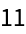#Function Repository Resource:

# MinSumPermutation

Find a permutation that minimizes a matrix sum

Contributed by: Wolfram Research
 ResourceFunction["MinSumPermutation"][m] finds a permutation p such that the quantity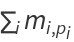for the matrix m is minimized.

## Details and Options

ResourceFunction["MinSumPermutation"] uses integer linear optimization to find the optimal matrix.

## Examples

### Basic Examples

Return the minimizing permutation:

 In:=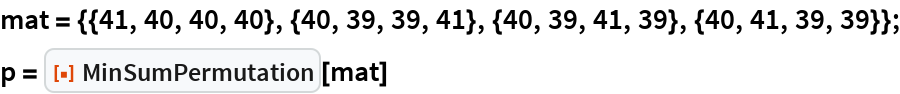Out=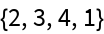The sum:

 In:=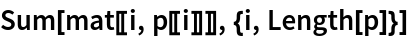Out=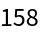### Scope

Use on a larger example:

 In:=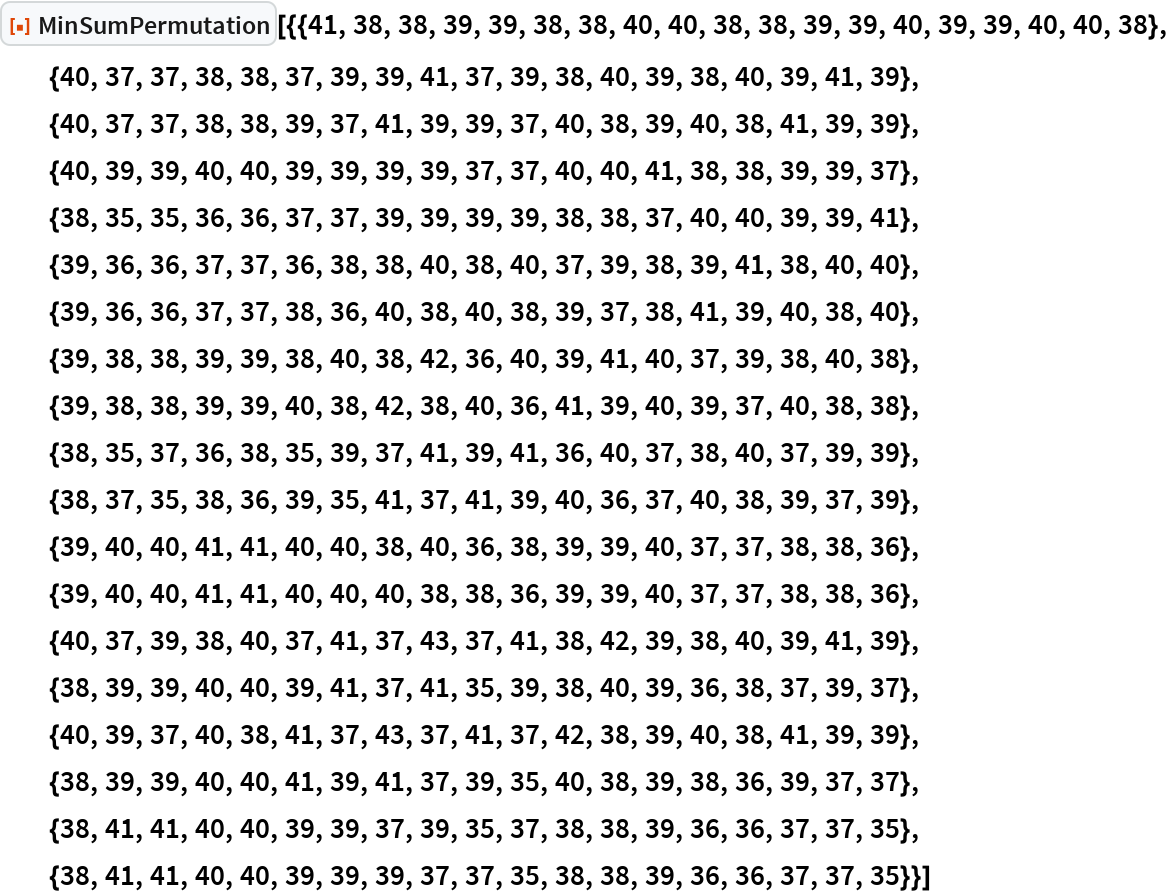Out=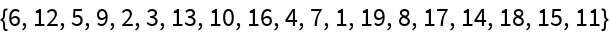### Possible Issues

For an m×n matrix with m>n, a solution might not exist: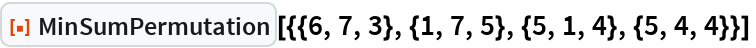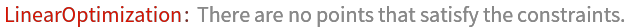Out=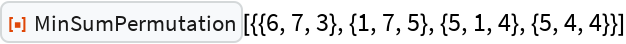If m<n, the result might not necessarily be a permutation:

 In:=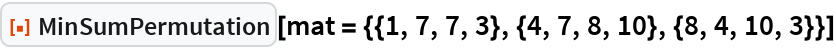Out=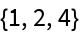In:=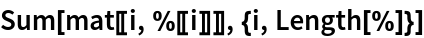Out=